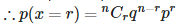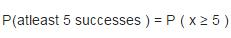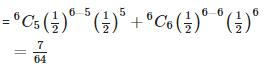# Test: Probability (CBSE Level) - 1

## 25 Questions MCQ Test Mathematics (Maths) Class 12 | Test: Probability (CBSE Level) - 1

Description
This mock test of Test: Probability (CBSE Level) - 1 for JEE helps you for every JEE entrance exam. This contains 25 Multiple Choice Questions for JEE Test: Probability (CBSE Level) - 1 (mcq) to study with solutions a complete question bank. The solved questions answers in this Test: Probability (CBSE Level) - 1 quiz give you a good mix of easy questions and tough questions. JEE students definitely take this Test: Probability (CBSE Level) - 1 exercise for a better result in the exam. You can find other Test: Probability (CBSE Level) - 1 extra questions, long questions & short questions for JEE on EduRev as well by searching above.
QUESTION: 1

### The conditional probability of an event E, given the occurrence of the event F is given by

Solution:

The conditional probability of an event E, given the occurrence of the event F is given by :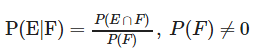QUESTION: 2

### A coin is tossed three times, if E : head on third toss , F : heads on first two tosses. Find P(E|F)

Solution:

S ={HHH,HHT,HTH,THH,HTT,THT,TTH,TTT}
E = {HHH,HTH,THH,TTH}
F = {HHH,HHT}
E∩F = {HHH}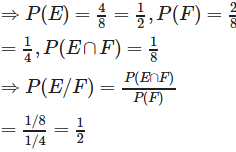QUESTION: 3

### Two cards are drawn at random and without replacement from a pack of 52 playing cards. Find the probability that both the cards are black.

Solution:

Required probability : P(BB) = P(B) X P(B/B) ……………{B means black card}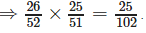QUESTION: 4

A laboratory blood test is 99% effective in detecting a certain disease when it is in fact, present. However, the test also yields a false positive result for 0.5% of the healthy person tested (i.e. if a healthy person is tested, then, with probability 0.005, the test will imply he has the disease). If 0.1 percent of the population actually has the disease, what is the probability that a person has the disease given that his test result is positive?

Solution:

Let
E1 and E2 are events that a person has disease and the person has not disease.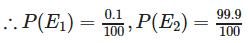Let A  = event that the test result is positive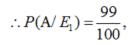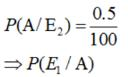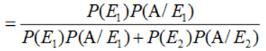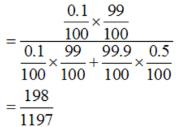QUESTION: 5

Suppose that two cards are drawn at random from a deck of cards. Let X be the number of aces obtained. Then the value of E(X) is

Solution:

The possible values of X are 0 , 1 and 2 .
As we know that pack of cards contains 4 aces.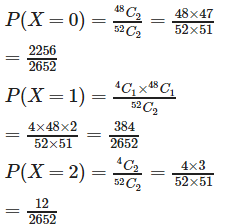Therefore , the probability distribution of X is :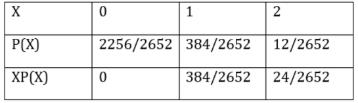Therefore required value of
E(X) is = 0+ 384/2652 + 24/2652 = 408/2652 = 2/13.

QUESTION: 6

The conditional probability of an event E, given the occurrence of the event F

Solution:

As the probability of any event always lies between 0 and 1. Therefore , 0 ≤ P (E|F) ≤ 1.

QUESTION: 7

A coin is tossed three times, if E : at least two heads , F : at most two heads. Find P(E|F)

Solution: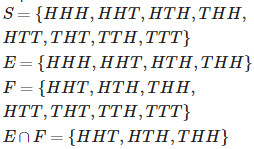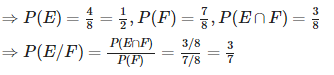QUESTION: 8

A box of oranges is inspected by examining three randomly selected oranges drawn without replacement. If all the three oranges are good, the box is approved for sale, otherwise, it is rejected. Find the probability that a box containing 15 oranges out of which 12 are good and 3 are bad ones will be approved for sale.

Solution:

Total oranges = 15., Good Oranges = 12. Let G stands for a good orange. Therefore , Required Probability = P(GGG) = P(G).P(G/G).P(G/GG)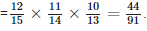QUESTION: 9

There are three coins. One is a two headed coin (having head on both faces),another is a biased coin that comes up heads 75% of the time and third is an unbiased coin. One of the three coins is chosen at random and tossed, it shows heads, what is the probability that it was the two headed coin ?

Solution:

Let
E1 , E2 and E3 and are events of selection of a two headed coin , biased coin and unbiased coin respectively.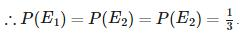Let A = event of getting head.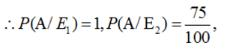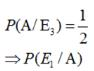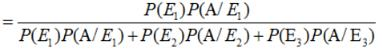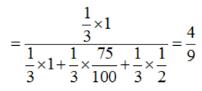QUESTION: 10

Which of the following conditions do Bernoulli trials satisfy?

Solution:

Bernoulli trials satisfies the finite number of independent trials .

QUESTION: 11

The conditional probability of an event E’s complement E’, given the occurrence of the event F

Solution:

As the total probability of an event is always 1 . therefore , P (E′|F) = 1 – P (E|F).

QUESTION: 12

A coin is tossed three times, E : at most two tails , F : at least one tail. Find P(E|F)

Solution: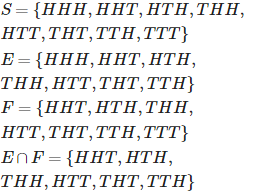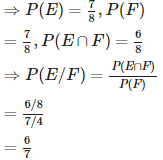QUESTION: 13

Given that the events A and B are such that P(A) = 1/2 , P (A ∪ B) = 3/5 and P(B) = p. Find p if they are mutually exclusive

Solution: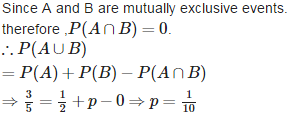QUESTION: 14

An insurance company insured 2000 scooter drivers, 4000 car drivers and 6000 truck drivers. The probability of an accidents are 0.01, 0.03 and 0.15 respectively. One of the insured persons meets with an accident. What is the probability that he is a scooter driver?

Solution:

Let
E1 , E2 and E3 and are events of selection of a scooter driver , car driver and truck driver respectively.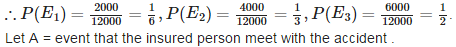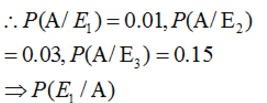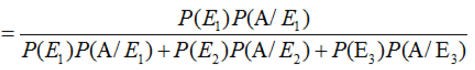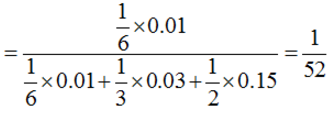QUESTION: 15

Which of the following conditions do Bernoulli trials satisfy?

Solution:

Bernoulli trials are true only when Each trial has exactly two outcomes: success or failure and the probability of success or failure remains the same in each trial.

QUESTION: 16

If E, F and G are events then P ((E ∪ F)|G) =

Solution:

If E, F and G are events then P ((E ∪ F)|G) represents the conditional probability of the given event . therefore P ((E ∪ F)|G) = P (E|G) + P (F|G) – P ((E ∩ F)|G) .

QUESTION: 17

A black and a red dice are rolled. Find the conditional probability of obtaining a sum greater than 9, given that the black die resulted in a 5.

Solution: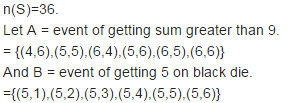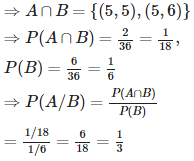QUESTION: 18

Given that the events A and B are such that P(A) = 1/2 P (A ∪ B) = 3/5 and P(B) = p. Find p if they independent.

Solution:

Since A and B are independent events. Therefore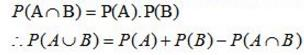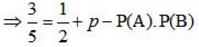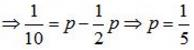QUESTION: 19

A random variable is a real valued function whose domain is the.

Solution:

A random variable is a real valued function whose domain is the sample space of a random experiment .

QUESTION: 20

A die is thrown 6 times. If ‘getting an odd number’ is a success, what is the probability of 5 successes?

Solution:

p = probability of success = 3/6 = ½ . q = probability of failure = 1 – p = 1 – ½ = ½ . let x be the number of successes , then x has the binomial distribution with : n = 6 , p = ½ , q = ½ .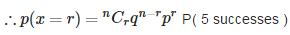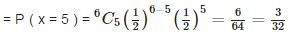QUESTION: 21

If E and F are events then P (E ∩ F) =

Solution:

If E and F are events then P (E ∩ F) = P (E) P (F|E), P (E) ≠ 0.

QUESTION: 22

A black and a red dice are rolled. Find the conditional probability of obtaining the sum 8, given that the red die resulted in a number less than 4.

Solution:

n(S)=36.
Let A = event of getting sum 8.
= {(2,6),(3,5),(4,4),(5,3),(6,2),}
And B = event of getting a number less than 4 on red die.
={(1,1),(2,1),(3,1),(4,1),(5,1),(5,1), (1,2),(2,2),(3,2),(4,2),(5,2),(6,2), (1,3),(2,3),(3,3),(4,3),(5,3),(6,3)}.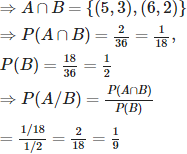QUESTION: 23

Let A and B be independent events with P (A) = 0.3 and P(B) = 0.4. Find P(A ∩ B)

Solution:

Let A and B be independent events with P (A) = 0.3 and P(B) = 0.4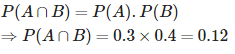QUESTION: 24

Let X be a random variable assuming values x1, x2,....,xn with probabilities p1, p2, ...,pn, respectively such that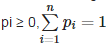. Mean of X denoted by μ is defined as

Solution:

Let X be a random variable assuming values x1, x2,....,xn with probabilities p1, p2, ...,pn, respectively such thatMean of X denoted by μ is defined as: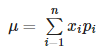QUESTION: 25

A die is thrown 6 times. If ‘getting an odd number’ is a success, what is the probability of at least 5 successes?

Solution:

p = probability of success = 3/6 = ½ .
q = probability of failure = 1 – p = 1 – ½ = ½
. let x be the number of successes , then x has the binomial distribution with :
n = 6 , p = ½ , q = ½ .